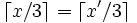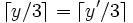# Mathematics of Sudoku

Mathematics of Sudoku

The general problem of solving Sudoku puzzles on n2 x n2 boards of n x n blocks is known to be NP-complete. This gives some vague indication of why Sudoku is hard to solve, but on boards of finite size the problem is finite and can be solved by a deterministic finite automaton that knows the entire game tree.

However, for a non-trivial starting board, the game tree is very large and so this method is not feasible. The problem of solving a puzzle that is known to have only one solution is in UP.

Solving Sudoku puzzles can be expressed as a graph colouring problem. The aim of the puzzle in its standard form is to construct a proper 9-colouring of a particular graph, given a partial 9-colouring. The graph in question has 81 vertices, one vertex for each cell of the grid. The vertices can be labelled with the ordered pairs , where x and y are integers between 1 and 9. In this case, two distinct vertices labelled by and are joined by an edge if and only if:or,or,andThe puzzle is then completed by assigning an integer between 1 and 9 to each vertex, in such a way that vertices that are joined by an edge do not have the same integer assigned to them.

A valid Sudoku solution grid is also a Latin square. There are significantly fewer valid Sudoku solution grids than Latin squares because Sudoku imposes the additional regional constraint. Nonetheless, the number of valid Sudoku solution grids for the standard 9x9 grid was calculated by Bertram Felgenhauer in 2005 to be 6,670,903,752,021,072,936,960, which is roughly the number of micrometers to the nearest star. This number is equivalent to 9! x 72^2 x 2^7 x 27,704,267,971, the last factor of which is prime. The result was derived through logic and brute force computation. The derivation of this result was considerably simplified by analysis provided by Frazer Jarvis and the figure has been confirmed independently by Ed Russell. A paper detailing the methodology of their analysis can be found at. The number of valid Sudoku solution grids for the 16x16 derivation is not known.

Of course, some of the 9x9 grids can easily be transformed into others; by relabelling the numbers, by rotating or reflecting the grid, and by permuting certain rows and columns. Ed Russell and Frazer Jarvis have counted the number of "essentially different" sudoku grids as 5,472,730,538: see the previous link for more details of the calculation.

Paul Muljadi discovers magic Sudoku, a Sudoku which contains at least one 3x3 normal magic square anywhere in the solution grid. Ed Russell creates 64 possible arrangements of magic Sudoku of five normal 3x3 magic squares in each.

The maximum number of givens that can be provided while still not rendering the solution unique, regardless of variation, is four short of a full grid; if two instances of two numbers each are missing and the cells they are to occupy are the corners of an orthogonal rectangle, there are two ways the numbers can be added. The inverse of this - the fewest givens that render a solution unique - is an unsolved problem, although the lowest number yet found for the standard variation without a symmetry constraint is 17, a number of which have been found by Japanese puzzle enthusiasts and 18 with the givens in rotationally symmetric cells.